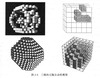cellular automata

CA

• ## matlab元胞自动机入门详解

万次阅读 多人点赞 2019-02-09 11:13:08
元胞自动机的初步理解 对元胞自动机的初步认识 元胞自动机（CA）是一种用来仿真局部规则和局部联系的方法。典型的元 胞自动机是定义在网格上的，每一个点上的网格代表一个元胞与一种有限的状 态。变化规则适用于每...
元胞自动机的初步理解

对元胞自动机的初步认识
元胞自动机（CA）是一种用来仿真局部规则和局部联系的方法。典型的元
胞自动机是定义在网格上的，每一个点上的网格代表一个元胞与一种有限的状
态。变化规则适用于每一个元胞并且同时进行。
元胞的变化规则&元胞状态
典型的变化规则，决定于元胞的状态，以及其（ 4 或 8 ）邻居的状态。
元胞自动机的应用
元胞自动机已被应用于物理模拟，生物模拟等领域。
元胞自动机的matlab编程
结合以上，我们可以理解元胞自动机仿真需要理解三点。一是元胞，在matlab中可以理解为矩阵中的一点或多点组成的方形块，一般我们用矩阵中的一点代表一个元胞。二是变化规则，元胞的变化规则决定元胞下一刻的状态。三是元胞的状态，元胞的状态是自定义的，通常是对立的状态，比如生物的存活状态或死亡状态，红灯或绿灯，该点有障碍物或者没有障碍物等等。

更新画面
动态的展示变化过程，需要掌握下面三条语句
h = imagesc(你的元胞矩阵)
在按照一定规则更行状态的循环中，
set(h,'cdata',你的更新后的元胞矩阵)
drawnow或者pause(时间间隔)

元胞自动机的有趣实例
生命游戏机
生命游戏是英国数学家约翰·何顿·康威在1970年发明的细胞自动机。它包括一个二维矩形世界，这个世界中的每个方格居住着一个活着的或死了的细胞。一个细胞在下一个时刻生死取决于相邻八个方格中活着的或死了的细胞的数量。通常情况，游戏的规则就是：当一个方格周围有2或3个活细胞时，方格中的活细胞在下一个时刻继续存活；即使这个时刻方格中没有活细胞，在下一个时刻也会“诞生”活细胞。
听说许多程序猿都喜欢玩这个
规则是：
¾ 对周围的 8 个近邻的元胞状态求和
¾ 如果总和为 2 的话，则下一时刻的状态不改变
¾ 如果总和为 3 ，则下一时刻的状态为 1
¾ 否则状态= 0
元胞的邻居定义通常有以下三种范式，这里采用第二种，认为其周围八个点为邻居。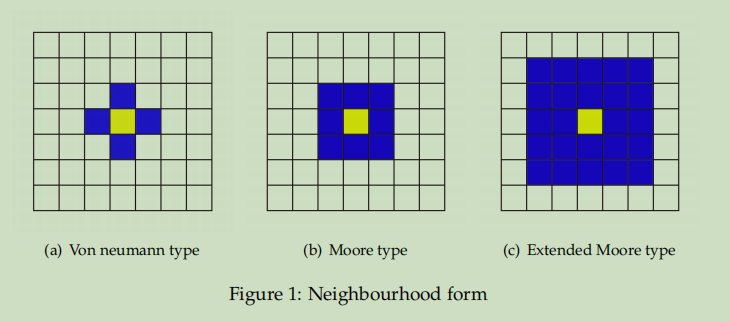简单仿真效果：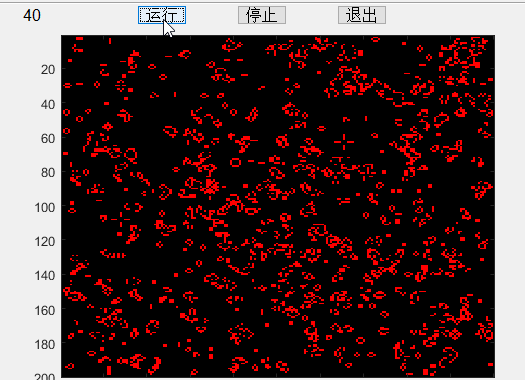全部代码如下
%% 设置GUI按键
plotbutton=uicontrol('style','pushbutton','string','运行', 'fontsize',12, 'position',[150,400,50,20], 'callback', 'run=1;');
erasebutton=uicontrol('style','pushbutton','string','停止','fontsize',12,'position',[250,400,50,20],'callback','freeze=1;');
quitbutton=uicontrol('style','pushbutton','string','退出','fontsize',12,'position',[350,400,50,20],'callback','stop=1;close;');
number = uicontrol('style','text','string','1','fontsize',12, 'position',[20,400,50,20]);
%% 元胞自动机设置
n=200;
%初始化各元胞状态
z = zeros(n,n);
sum = z;
cells = (rand(n,n))<.6;
% 建立图像句柄
imh = image(cat(3,cells,z,z));
set(imh, 'erasemode', 'none')
% 元胞更新的行列数设置
x = 2:n-1;
y = 2:n-1;
% 主事件循环
stop= 0; run = 0;freeze = 0;
while stop==0
if run==1
% 计算邻居存活的总数
sum(x,y) = cells(x,y-1) + cells(x,y+1) + cells(x-1, y) + cells(x+1,y)...
+ cells(x-1,y-1) + cells(x-1,y+1) + cells(x+1,y-1) + cells(x+1,y+1);
% 按照规则更新
cells = (sum==3) | (sum==2 & cells);
set(imh, 'cdata', cat(3,cells,z,z) )
stepnumber = 1 + str2double(get(number,'string'));
set(number,'string',num2str(stepnumber))
end
if freeze==1
run = 0;
freeze = 0;
end
drawnow
end

扩散
规则，先把中间点置为1，每一时间步对每一点，如果周围
八个点和为偶数，则变为0，为奇数则变为 1
效果：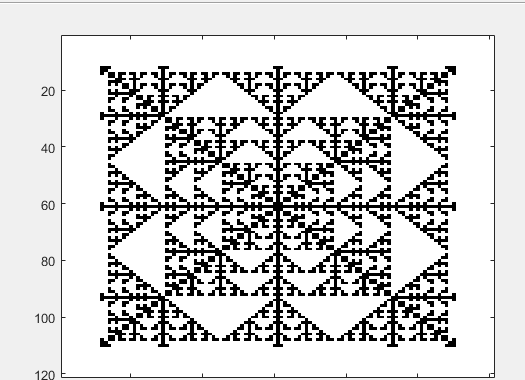代码：
% 颜色控制
Map = [1 1 1; 0 0 0];
colormap(Map);
% 设置网格大小
S = 121;
L = zeros(S);
% 把中间一个数设置为 1 作为元胞种子
M = (S+1)/2;
L(M, M) = 1;
Temp = L;
imagesc(L);
% 计算层数
Layer = (S-1)/2 + 1;

for t=2:Layer
for x=M-t+1:M+t-1
if x==M-t+1 || x==M+t-1

for y=M-t+1:M+t-1
SUM = 0;
for m=-1:1
for n=-1:1
if x+m>0 && x+m<=S && y+n>0 && y+n<=S
SUM = SUM + L(x+m, y+n);
end
end
end
SUM = SUM - L(x, y);
Temp(x, y) = mod(SUM, 2);
end

else
y = M-t+1;
SUM = 0;
for m=-1:1
for n=-1:1
if x+m>0 && x+m<=S && y+n>0 && y+n<=S
SUM = SUM + L(x+m, y+n);
end
end
end
SUM = SUM - L(x, y);
Temp(x, y) = mod(SUM, 2);

y = M+t-1;
SUM = 0;
for m=-1:1
for n=-1:1
if x+m>0 && x+m<=S && y+n>0 && y+n<=S
SUM = SUM + L(x+m, y+n);
end
end
end
SUM = SUM - L(x, y);
Temp(x, y) = mod(SUM, 2);
end
end
L = Temp;
imagesc(L);
% 速度控制
pause(0.2);
end

双车道交通流仿真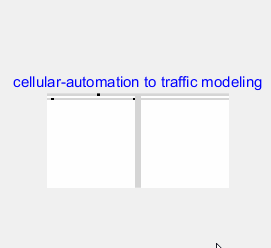这个稍微难一点
脚本
% 车流密度不变下的双向两车道仿真（T 字形路口）
% v:平均速度，d:换道次数（1000 次）p:车流密度
nl = 80 ;% 车道长度（偶数）
nc = 2; % nc:双向车道数目
dt= 0.01; % 仿真步长时间
fp = 20; % 车道入口处新进入车辆的概率（列向量）
nt=10000;% 仿真步长数目
chance=0.5;
chance1=0.5;
[ v, d, p ] = multi_driveway_with_crossroad_exit ( nl,nc,dt,fp,nt,chance,chance1);


规则&状态转移函数
function [ v, d, p ] = multi_driveway_with_crossroad_exit( nl,...
nc,dt,fp,nt,chance,chance1)
% fp:车道入口处新进入车辆的概率向量（2,3,5 车道）——输入参数
% chance:交叉口处车辆行为的概率向量(5 车道右转,3车道右转）——输入参数
%构造元胞矩阵
B=ones(nc+1+nl/2,nl+3);
%不可行车道
B(nc/2+1,[1:nl/2 nl/2+4:nl+3])=1.2;
B(nc+2:nc+1+nl/2,[1:nl/2 nl/2+4:nl+3])=1.2;
%初始化仿真元胞状态（1 为无车，0 为有车）
bb1=B([1:nc/2 nc/2+2:nc+1],:);bb2=B(:,nl/2+3);bb3=B(:,nl/2+1);
bb1(bb1~=0)=1;
bb2(bb2~=0)=1;
bb3(bb3~=0)=1;
B([1:nc/2 nc/2+2:nc+1],:)=bb1;B(:,nl/2+3)=bb2;B(:,nl/2+1)=...
bb3;B(1:nc+1,nl/2+1:nl/2+3)=1;
B(1:nc/2,end)=0;B(nc/2+2:nc+1,1)=0;B(end,nl/2+3)=0;
%显示初始交通流图
figure();
H=imshow(B,[]);
set(gcf,'position',[241 132 560 420]) ;%241 132 560 420
set(gcf,'doublebuffer','on'); %241
title('cellular-automation to traffic modeling','color','b');
%初始化化存储元胞上车辆状态的矩阵
S(1:nc*2+2,nl/2-2) = 0;
Q(1:nc*2+2,1:2) = 0;
C=zeros(nc+1,3);
%初始化换道频率、平均速度、车流密度相关变量
av(1:nt) = 0;
ap(1:nt) = 0;
s = 1;flag= 0;flag1=0;%flag、flag1 用于标示小区出口的车是否为左转车辆
flag2=0;
for n = 1:nt
%六个路段的长度。
A=[
B(1:nc/2,nl/2 :-1:1);
B(nc/2+2:nc+1,1:nl/2);
B(1:nc/2,nl+3:-1:nl/2+4);
B(nc/2+2:nc+1,nl/2+4:nl+3);
B(nc+1+nl/2:-1:nc+2,nl/2+3)';
B(nc+2:1:nc+1+nl/2,nl/2+1)'
];
c=B(1:nc+1,nl/2+1:nl/2+3);
%确定前 n-2 个车辆的状态
S(:,:) = 0;
S(A(:,1:end-2)==0&A(:,2:end-1)==1&A(:,3:end)==1)=2;%快速行驶的车
S(A(:,1:end-2)==0&A(:,2:end-1)==0)=3;%停车的车
S(A(:,1:end-2)==0&A(:,2:end-1)==1&A(:,3:end)==0)=1;%慢速行驶的车
%确定最后两个元胞的状态
Q(:,:)= 0;
Q(A(:,end-1)==0&A(:,end)==0) = 3;
Q(A(:,end-1)==0&A(:,end)==1) = 1;
if c(3,1)==0
if rand<chance1
flag2=1;
c(3,1)=1;
end
end
if A(1,end)==0
Q(1,end)=1;
end
if A(4,end)==0
Q(4,end)=1;
end
if A(6,end)==0
Q(6,end)=1;
end
if rem(floor(n/50),2)==0 %此时左右向为绿灯
if A(2,end)==0
if c(nc/2+2:nc+1,1)==0
Q(2,end)=3;
else
Q(2,end)=1;
end
end
if A(3,end)==0
if c(1,3)==0
Q(3,end)=3;
else
Q(3,end)=1;
end
end
%按照既定规则行驶（5 车道右转）
if A(5,end)==0
if flag==0
if rand<chance %路口车右转
if c(nc/2+2:nc+1,:)==1
Q(5,end)=1;
else
Q(5,end)=3;
end
end
else %第一辆车为左转车，需要等待
end
end
if c(1,2)==0
if c(1,1)==1%3道口左转的思路：规避。
C(1,2)=1;
else
C(1,2)=3;
end
if c(2,1)==0
C(1,2)=3;
end
end
if c(1,3)==0
if c(1,2)==1
C(1,3)=1;
else
C(1,3)=3;
end
end
if c(3,1)==0
if c(3,2)==1
C(3,1)=1;
else
C(3,1)=3;
end
end
if c(3,2)==0
if c(3,3)==1
C(3,2)=1;
else
C(3,2)=3;
end
end
if rem(n,20)==0&&c(3,2)==0%小区出来的车还遗留在路口，特殊处理先行
if c(2,1)==1
C(3,2)=5; %特殊的等待状态（小区出来的车）
else
C(3,2)=3;
end
end
if c(2,1)==0
if A(1:nc/2,1)==0
C(2,1)=3;
else
C(2,1)=1;
end
end
if c(1,1)==0
if A(1,1)==0
C(1,1) = 3;
else
C(1,1) = 1;
end
end
if c(3,3)==0
if A(nc*3/2+1:2*nc,1)==0
C(3,3) = 3;
else
C(3,3) = 1;
end
end
else %此时小区出入向为绿灯
Q(2,end)=3;Q(3,end)=3;
if c(3,2)==0
if flag1==1
if c(2,1)==1
C(3,2)=5;flag1=0;
else
C(3,2)=3;
end
else
if c(3,3)==1
C(3,2)=1;
else
C(3,2)=3;
end
end
end
if c(2,1)==0
if A(1:nc/2,1)==1&&c(1,1)==1
C(2,1)=1;
else
C(2,1)=3;
end
end
if A(5,end)==0
if flag==0
if rand<chance
if c(nc/2+2:nc+1,:)==1
Q(5,end)=1;
else
Q(5,end)=3;
end
else
if c(nc/2+2:nc+1,1)==1&&c(nc/2+2:nc+1,2)==1
Q(5,end)=5;flag=0;flag1=1; %小区的左转前进，用以区分右转车辆
else
Q(5,end)=3;flag=1;
end
end
else
if c(nc/2+2:nc+1,1)==1&&c(nc/2+2:nc+1,2)==1
Q(5,end)=5;flag=0;flag1=1; %小区的左转前进，用以区分右转车辆
else
Q(5,end)=3;flag=1;
end
end
end
if c(1,2)==0
if c(1,1)==1
C(1,2)=1;
else
C(1,2)=3;
end
end
if c(1,3)==0
if c(1,2)==1
C(1,3)=1;
else
C(1,3)=3;
end
end
if c(3,1)==0
if c(3,2)==1
C(3,1)=1;
else
C(3,1)=3;
end
end
if c(1,1)==0
if A(1:nc/2,1)==0
C(1,1) = 3;
else
C(1,1) = 1;
end
end
if c(3,3)==0
if A(nc*3/2+1:2*nc,1)==0
C(3,3) = 3;
else
C(3,3) = 1;
end
end
end
%获得所有元胞上车辆的状态
Acc = [ S Q ];
%根据当前状态改变元胞位置
%路口附近的车辆的行驶控制
if C(3,2)==5
c(2,1)=0;
c(3,2)=1;
flag=0;
C(3,2)=0;
elseif C(3,2)==1
c(3,3)=0;
c(3,2)=1;
C(3,2)=0;
end
if C(2,1)==1
A(1,1)=0;
c(2,1)=1;
C(2,1)=0;
end
if Acc(3,end)==1
c(1,3)=0;
A(3,end)=1;
Acc(3,end)=0;
end
if Acc(2,end)==1
c(3,1)=0;
A(2,end)=1;
Acc(2,end)=0;
end
if C(3,1)==1
c(3,2)=0;
c(3,1)=1;
C(3,1)=0;
end
if C(1,3)==1
c(1,2)=0;
c(1,3)=1;
C(1,3)=0;
end
if C(1,2)==1
c(1,1)=0;
c(1,2)=1;
C(1,2)=0;
end
if C(1,1)==1
A(1,1)=0;
c(1,1)=1;
C(1,1)=0;
end
if C(3,3)==1
A(4,1)=0;
c(3,3)=1;
C(3,3)=0;
end
%慢速运行车辆向前走 1 格
A( Acc(:,1:end)==1 )=1;
A( [ zeros(nc*3,1) Acc(:,1:end-1)]==1 ) = 0;
%高速运行车辆向前走 2 格
A( Acc(:,1:end)==2) = 1;
A( [ zeros(nc*3,2) Acc(:,1:end-2)]==2) = 0;
if Acc(1,1)==1||Acc(1,1)==2
A(1,1)=1;
end
if Acc(4,1)==1||Acc(4,1)==2
A(4,1)=1;
end
if Acc(5,end)==5
c(3,2)=0;flag=0;
A(5,end)=1;
elseif Acc(5,end)==1
c(3,3)=0;
A(5,end)=1;
end
if Acc(3,end)==1
c(1,3)=0;
A(3,end)=1;
end
if Acc(2,end)==1
c(3,1)=0;
A(2,end)=1;
end
if Acc(4,1)==1||Acc(4,1)==2
A(4,1)=1;
end
if Acc(1,1)==1||Acc(1,1)==2
A(1,1)=1;
end
%计算平均速度、换道频率、车流密度等参数
%获得运行中的车辆数目 N
matN = A<1;
N = sum(sum(matN));
%获得运行中的车辆速度之和 V
E = S((S==1)|(S==2));
V = sum(E);
%计算此时刻的车流密度并保存
ap(n) = N/( (nc*3)*(nl/2)+9 );
%计算此时刻的平均速率并保存
if(N~=0&&n>nl/2)
av(s) = V/N;
s = s+1;
end
%在车道入口处随机引入新的车辆
A([2;3;5],1)=(round(fp.*rand(3,1))&A([2;3;5],1));
A(A~=0)=1;
if flag2==1
A(6,1)=0;
flag2=0;
end
%将新的车辆加入元胞矩阵中
B(1,1:nl/2)=A(1:nc/2,end:-1:1);
B(3,1:nl/2)=A(nc/2+1:nc,:);
B(1,nl/2+4:nl+3)=A(nc+1:nc*3/2,end:-1:1);
B(3,nl/2+4:nl+3)=A(nc*3/2+1:2*nc,:);
B(nc+2:nc+1+nl/2,nl/2+3)=A(2*nc+1,end:-1:1)';
B(nc+2:nc+1+nl/2,nl/2+1)=A(3*nc,:)';
B(1:3,nl/2+1:nl/2+3)=c(:,:);
%显示交通流图
set(H,'CData',B);
%计算这个时间段每个时间点的指标（速度与车流量）。
p = mean(ap);
v = sum(av)/s;
disp([v,p])
%仿真步长
pause(dt);
end
end



展开全文• 故其分类难度也较大，自元胞自动机产生以来，对于元胞自动机分类的研究就是元胞自动机的一个重要的研究课题和核心理论，在基于不同的出发点，元胞自动机可有多种分类，其中，最具影响力的当属S. Wolfram在80年代初做...
• ## MATLAB演示元胞自动机算法

万次阅读 多人点赞 2019-03-03 22:59:45
一、元胞自动机理论 元胞自动机与格子理论是一个非常好的模型，许多复杂的问题都可以通过它来建立模型，下面就简要介绍一下。 元胞自动机 实质上是定义在一个具有离散、有限状态的元胞组成的元胞空间上，并按照...
一、元胞自动机理论

元胞自动机与格子理论是一个非常好的模型，许多复杂的问题都可以通过它来建立模型，下面就简要介绍一下。

元胞自动机

实质上是定义在一个具有离散、有限状态的元胞组成的元胞空间上，并按照一定的局部规则，在离散的时间维度上演化的动力学系统。

元胞

元胞又可称为单元、细胞，是元胞自动机的最基本的组成部分。

元胞具有以下特点：

元胞自动机最基本的单元。
元胞有记忆贮存状态的功能。
所有元胞状态都按照元胞规则不断更新。
演化规则

中心元胞的下一个状态由中心元胞的当前状态和其邻居的当前状态按照一定的规则确定。

二、森林火灾的演示

下面就用MATLAB来演示森林火灾，以便更好地理解元胞自动机理论。

森林火灾的元胞自动机模型有三种状态：空位，燃烧着的树木及树木。则某元胞下一时刻状态由该时刻本身的状态和周围四个邻居的状态以一定的规则确定，规则如下：

如果某树木元胞的4个邻居有燃烧着的，那么该元胞下一时刻的状态是燃烧着的。
一个燃烧着的元胞在下一时刻变成空位。
所有树木元胞以一个低概率开始燃烧（模拟闪电引起的火灾）
所有空元胞以一个低概率变成树木（以模拟新的树木的生长）
n = 300;     %元胞矩阵大小
Plight = .000005; Pgrowth = .01;
UL = [n 1:n-1];
DR = [2:n 1];
veg = zeros(n,n);        %初始化
% The value of veg:
% empty == 0
% burning == 1
% green == 2
imh = image(cat(3,veg,veg,veg));
for i = 1:3000
sum = (veg(UL,:) == 1) + (veg(:,UL) == 1) + (veg(DR,:) == 1) + (veg(:,DR) == 1);
%根据规则更新森林矩阵：树 = 树 - 着火的树 + 新生的树
veg = 2 * (veg == 2) - ( (veg == 2) & (sum > 0 | (rand(n,n) < Plight)) ) + 2 * ( (veg == 0) & rand(n,n) < Pgrowth);
set(imh, 'cdata', cat(3, (veg == 1), (veg == 2), zeros(n)) )
drawnow
pause(0.01)
end


运行的结果如下，可以通过调整闪电和树木生长的概率来观察不同的结果。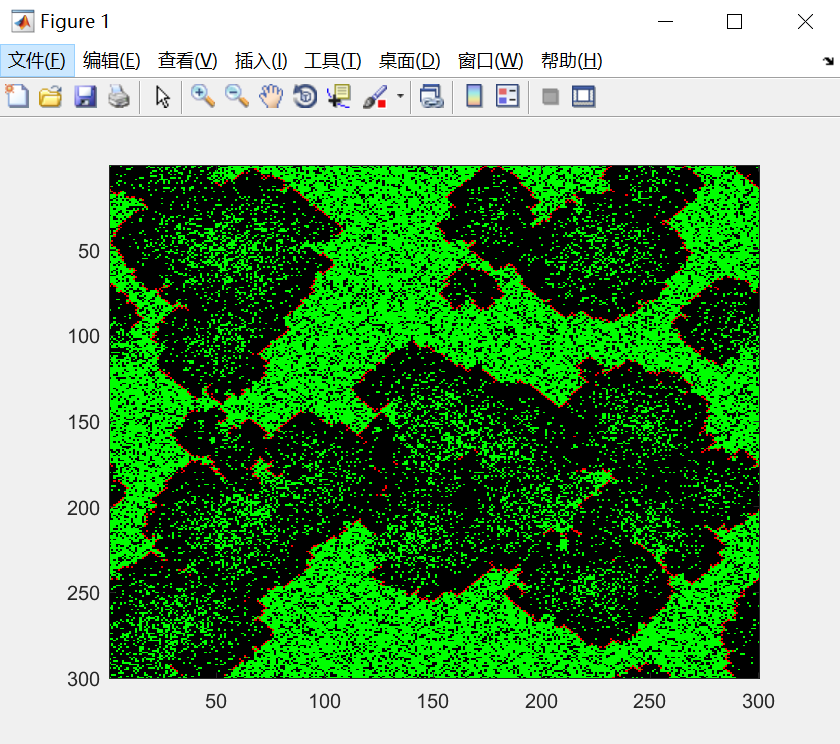如果你觉得有帮助，不妨点个赞哦~

展开全文Matlab
• 元胞自动机ppt，课程比较贵，这是里面元胞自动机部分的ppt，讲解清楚，看了会懂大致想法
• 元胞自动机及其在各个方面的应用，首先介绍元胞自动机的内容，后面关于复杂性做了深入的研究，背景部分讲的充实。
• 元胞自动机Python代码代码，打开即可使用，是已经调试好的代码，下载之后即可使用。
• 元胞自动机(Cellular Automaton, 缩写CA)是离散的动力学系统, 用简单规则来控制系统中的局部相互作用, 从而实现模拟复杂世界。此C语言代码可以画出一维元胞自动机的规则时空图。
• 元胞自动机模型，包括生命游戏演化规则，蚂蚁公路，格子气自动机，应用元胞自动机解决交通问题等
• 元胞自动机（CA）是一种用来仿真局部规则和局部联系的方法。典型的元胞自动机是定义在网格上的，每一个点上的网格代表一个元胞与一种有限的状态。变化规则适用于每一个元胞并且同时进行。典型的变化规则，决定于元胞...
• 交通流元胞自动机仿真平台V3.0 交通流元胞自动机仿真平台V3.0 交通流元胞自动机仿真平台V3.0 交通流元胞自动机仿真平台V3.0
• 元胞自动机(Cellular Automata)，简称CA，也有人译为细胞自动机、点格自动机、分子自动机或单元自动机)。是一时间和空间都离散的动力系统。散布在规则格网 (Lattice Grid)中的每一元胞(Cell)取有限的离散状态，遵循...
• 元胞自动机的Matlab代码.元胞自动机的Matlab代码.元胞自动机的Matlab代码.
• 内含多篇元胞自动机的经典文献资料，并附有实例支撑材料以及代码，适合于元胞自动机初学、深入学习。数学建模学习等...